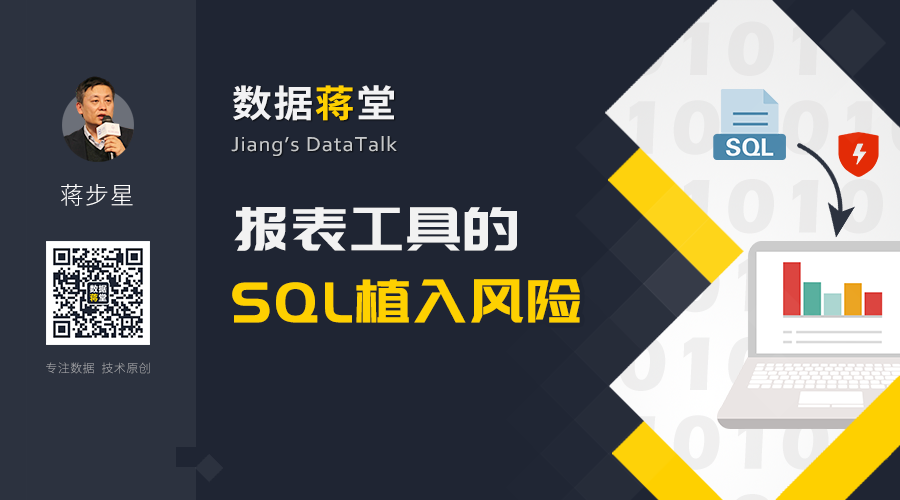# 报表工具的SQL植入风险SELECT … FROM T WHERE \${w}

1=0 UNION SELECT … FROM user

SELECT … FROM T WHERE 1=0 UNION SELECT … FROM user

SELECT … FROM T WHERE (\${w})

SELECT … FROM T WHERE (1=0 UNION SELECT … FROM user)

1=0) UNION SELECT … FROM user WHERE (1=1

SELECT … FROM T WHERE (1=0) UNION SELECT … FROM user WHERE (1=1)

SELECT … FROM T WHERE (\${w}) OR \${w}

SELECT … FROM T WHERE (1=0) UNION SELECT … FROM user WHERE (1=1) OR 1=0) UNION SELECT … FROM user WHERE (1=1